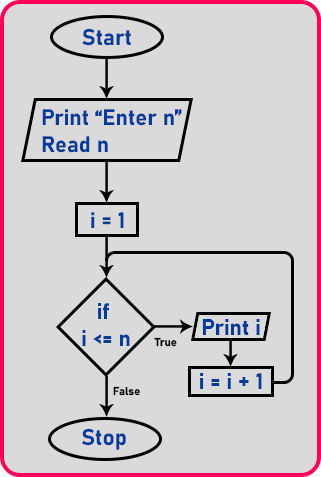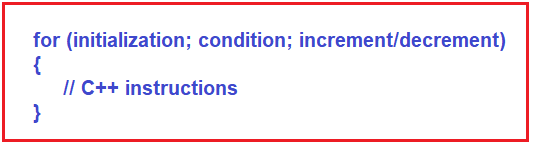# For Loop in C++

## For Loop in C++ with Examples

In this article, I am going to discuss the For loop in C++ Language with Examples. Please read our previous articles, where we discussed Do While loop in C++ with Examples. At the end of this article, you will understand what is for loop and when and how to use for loop in C++ program with examples.

##### For Loop in C++:

For loop is the type of loop that is also used for repetition and it is the most commonly used loop. If we know the number of times, we want to execute some set of statements or instructions, then we should use for loop. For loop is known as Counter Controlled loop. Whenever counting is involved for repetition, then we need to use for loop.

Let’s take an example and try to understand what does it mean by counting. Suppose you are preparing coffee. You don’t know how to prepare coffee; somebody has given you instruction and you’re following it. You are making coffee for 4 people.

Now the question is how much sugar you should add? You should add the sugar and mix it, again add the sugar and mix and so on until coffee becomes sweet enough. So, how many times you should add sugar to coffee? It has no fixed answer because you will add sugar to coffee until it becomes sweet enough. So, this is not based on counting.

Now somebody gives you instructions that add 4 tablespoons of sugar to the coffee. So, this is based on counting. There are many examples in daily life. We do things for a fixed number of times.

Note: So, when you have to repeat the steps based on counting, then use for loop.

Let us take the example which we have discussed in the previous article by using a while and do-while loop for printing numbers up to some given number. But here we do that example by using for loop.

##### For Loop Flowchart:First, we will take the input as far as we want to print the number. So, we want to print numbers to a certain point. For that, we need a counter, so here we have ‘i’ as a counter. And we have initialized ‘i’ to 1. So ‘i’ starts from one onwards.

Then condition will check ‘if (i <= n)’ (n is the input). If the condition is true then print the value of ‘i’ and modify ‘i’ as ‘i = i + 1’. Again, the condition will be checked, if it is true then repeat the same steps which we discussed above, or if it is false the loop will be stopped, and control will come out of the loop.

##### For loop syntax in C++

The for loop is a repetitive, structure that allows the execution of instructions a specific amount of time. It has four stages.

1. Loop initialization
2. Condition evaluation
3. Execution of instruction
4. Increment/Decrement

Now let’s have a look at the for loop syntax:###### Explanation:
1. Loop Initialization: Loop initialization happens only once while executing the for loop for the first time, which means that the initialization part of for loop only executes once.
2. Condition Evaluation: Conditions in for loop are executed for each iteration and if the condition is true, it executes C++ instruction if false it comes out of the loop.
3. Execution of Instruction: After the condition is executed, control comes to the loop body i.e. C++ instructions and it gets executed.
4. Increment/Decrement: After executing the C++ instructions for loop increment/decrement part of for loop executed and again it will go to the condition evaluation stage.

So why there are 3 parts? Because in for loop, first, we have to initialize the counter then condition, and then the increment/decrement of the counter so that the loop execute statements again and again. In for loop, Initialization, condition, and addition / updation are together in one place. Now let us see this in the code part.

##### Program to print numbers from 1 to n using for loop in C++:
```#include <iostream>
using namespace std;
int main()
{
int n;
cout << "Enter number:" << endl;
cin >> n;
cout << endl;
for (int i = 1; i <= n; i++)
{
cout << i << endl;
}
return 0;
}
```
###### Output:In for loop, we can skip initialization, we can initialize a variable before for loop in the program. So, Initialization is optional in for loop.

##### Infinite Loop in C++:

We can place the increment/decrement statement in the body part. If we don’t give any increment/decrement statement in for loop or in the body then what happens? It will be an infinite loop without any updated statement. Infinite is a non-ending loop. Let’s see the example:

```#include <iostream>
using namespace std;
int main()
{
int n, i = 1;
for (; i <= n;)
{
cout << "Hello C++" << endl;
}
return 0;
}
```
###### Output:You can see that it will go on printing “Hello C++” because here counter is not updated and the termination condition will never reach so it will continue printing “Hello C++” until you exit the program.

##### Can we run for loop without condition?

Let us see this with an example:

```#include <iostream>
using namespace std;
int main()
{
int n, i = 1;
for (;;)
{
cout << i << " Hello C++" << endl;
i++;
}
return 0;
}
```
###### Output:Again, it will be an infinite loop. Because this time we have written increment/decrement statement but haven’t mentioned any termination condition in for loop. So here ‘i’ value keeps increasing and printing “Hello C++”.

##### We can write custom if statement inside for loop as:
```#include <iostream>
using namespace std;
int main()
{
int n, i = 1;
for (;;)
{
cout << i << " Hello C++" << endl;
i++;
if (i > 5)
break;
}
return 0;
}
```
###### Output:##### Nested for Loop in C++:

In nested for loop one or more for loops can be included in the body of the loop. In the nested for loop, the number of iterations will be equal to the number of iterations in the outer loop multiplied by the number of iterations in the inner loop. The syntax to use nested for loop is given below.##### Program to understand nested for loop in C++:
```#include <iostream>
using namespace std;
int main ()
{
int i;	//for outer loop counter
int j;	//for inner loop counter
for (i = 1; i <= 5; i++)
{
for (j = 1; j <= 10; j++)
{
cout << j;
}
cout << "\n";
}
return 0;
}
```
###### Output: